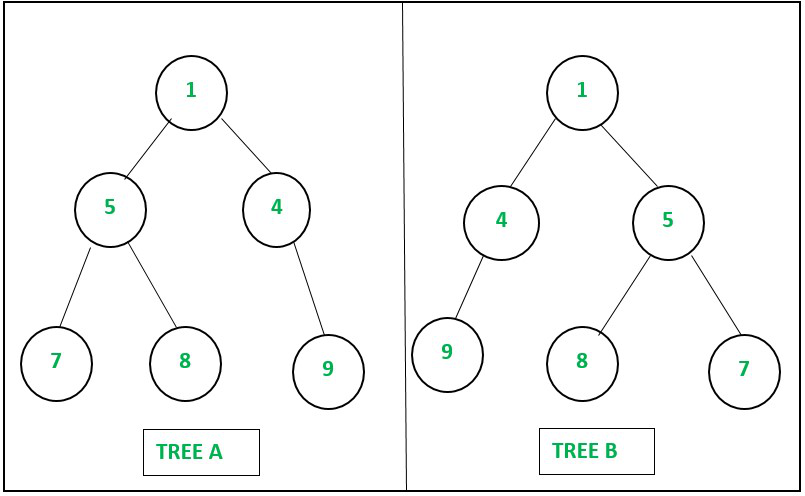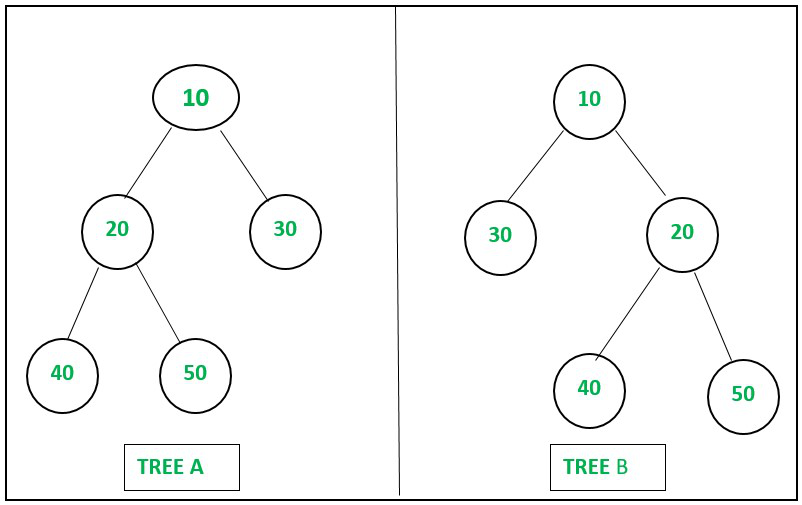Related Articles

# Check if two binary trees are mirror | Set 3

• Last Updated : 15 Jul, 2021

Given two arrays, A[] and B[] consisting of M pairs, representing the edges of the two binary trees of N distinct nodes according to the level order traversal, the task is to check if trees are the mirror images of each other.

Examples:

Input: N = 6, M = 5, A[] = {{1, 5}, {1, 4}, {5, 7}, {5, 8}, {4, 9}}, B[] = {{1, 4}, {1, 5}, {4, 9}, {5, 8}, {5, 7}}
Output: Yes
Explanation:Example 1

Input: N = 5, M = 4, A[] = {{10, 20}, {10, 30}, {20, 40}, {20, 50}}, B[] = {{10, 30}, {10, 20}, {20, 40}, {20, 50}}
Output: No
Explanation:Example 2

Set 1 and Set 2 of this article have been discussed in previous articles.

Approach: The given problem can be solved using Map and Set data structures. Follow the steps below to solve the problem:

• Initialize two, maps of vectors say T1 and T2 to store the adjacency list of trees A and B respectively.
• Initialize a set say St to store all the unique nodes’ values.
• Traverse the array A[] using the variable i, and performing the following steps:
• Push the value A[i] into the vector T1[A[i]] and then append the A[i] and A[i] to the set St.
• As the edges are given according to the level order traversal, therefore in tree A for all nodes, the left child was inserted first and then the right child was inserted.
• Traverse the array B[] in reverse order using the variable i and performing the following steps:
• Push the value B[i] into the vector T2[B[i]] and then append the B[i] and B[i] to the set St.
• As array B[] is traversed in reverse, therefore in the tree B for all nodes, the right child was inserted first and then the left child was inserted.
• Now iterate over the of set St and check if the vectors of children of the current node in tree A are not equal to the vectors of children of the current node in tree B, then print “No” as the answer and then return.
• Finally, if none of the above cases satisfy, then print “Yes” as the answer.

Below is the implementation of the above approach:

## C++

 `// C++ program for the above approach``#include ``using` `namespace` `std;` `// Function to check whether two binary``// trees are mirror image of each other``// or not``string checkMirrorTree(``int` `N, ``int` `M,``                       ``int` `A[], ``int` `B[])``{``    ``// Stores the adjacency list``    ``// of tree A``    ``map<``int``, vector<``int``> > T1;` `    ``// Stores the adjacency list``    ``// of tree B``    ``map<``int``, vector<``int``> > T2;` `    ``// Stores all distinct nodes``    ``set<``int``> st;` `    ``// Traverse the array A[]``    ``for` `(``int` `i = 0; i < M; i++) {` `        ``// Push A[i] in the``        ``// vector T1[A[i]]``        ``T1[A[i]].push_back(A[i]);` `        ``// Insert A[i] in the``        ``// set st``        ``st.insert(A[i]);` `        ``// Insert A[i] in the``        ``// set st``        ``st.insert(A[i]);``    ``}` `    ``// Traverse the array B[] in``    ``// reverse``    ``for` `(``int` `i = M - 1; i >= 0; i--) {` `        ``// Push B[i] in the``        ``// vector T2[B[i]]``        ``T2[B[i]].push_back(B[i]);` `        ``// Insert B[i] in the``        ``// set st``        ``st.insert(B[i]);` `        ``// Insert B[i] in the``        ``// set st``        ``st.insert(B[i]);``    ``}` `    ``// Iterate over the set st``    ``for` `(``auto` `node : st) {` `        ``// If vector T1[node] is``        ``// not equals to T2[node]``        ``if` `(T1[node] != T2[node])``            ``return` `"No"``;``    ``}` `    ``// Return "Yes" as``    ``// the answer``    ``return` `"Yes"``;``}` `// Driver Code``int` `main()``{``    ``// Given Input``    ``int` `N = 6;``    ``int` `M = 5;` `    ``int` `A[] = {``        ``{ 1, 5 }, { 1, 4 }, { 5, 7 }, { 5, 8 }, { 4, 9 }``    ``};``    ``int` `B[] = {``        ``{ 1, 4 }, { 1, 5 }, { 4, 9 }, { 5, 8 }, { 5, 7 }``    ``};` `    ``// Function Call``    ``cout << checkMirrorTree(N, M, A, B);` `    ``return` `0;``}`

## Python3

 `# Py program for the above approach` `# Function to check whether two binary``# trees are mirror image of each other``# or not``def` `checkMirrorTree(N, M, A, B):``  ` `    ``# Stores the adjacency list``    ``# of tree A``    ``T1 ``=` `[[] ``for` `i ``in` `range``(``100``)]` `    ``# Stores the adjacency list``    ``# of tree B``    ``T2 ``=` `[[] ``for` `i ``in` `range``(``100``)]` `    ``# Stores all distinct nodes``    ``st ``=` `{}` `    ``# Traverse the array A[]``    ``for` `i ``in` `range``(M):``      ` `        ``# Push A[i] in the``        ``# vector T1[A[i]]``        ``T1[A[i][``0``]].append(A[i][``1``])` `        ``# Insert A[i] in the``        ``# set st``        ``st[A[i][``0``]] ``=` `1` `        ``# Insert A[i] in the``        ``# set st``        ``st[A[i][``1``]] ``=` `1``        ` `    ``# Traverse the array B[] in``    ``# reverse``    ``for` `i ``in` `range``(M ``-` `1``, ``-``1``, ``-``1``):``        ``# Push B[i] in the``        ``# vector T2[B[i]]``        ``T2[B[i][``0``]].append(B[i][``1``])` `        ``# Insert B[i] in the``        ``# set st``        ``st[B[i][``0``]] ``=` `1` `        ``# Insert B[i] in the``        ``# set st``        ``st[B[i][``1``]] ``=` `1` `    ``# Iterate over the set st``    ``for` `node ``in` `st:` `        ``# If vector T1[node] is``        ``# not equals to T2[node]``        ``if` `(T1[node] !``=` `T2[node]):``            ``return` `"No"` `    ``# Return "Yes" as``    ``# the answer``    ``return` `"Yes"` `# Driver Code``if` `__name__ ``=``=` `'__main__'``:``    ``# Given Input``    ``N ``=` `6``    ``M ``=` `5` `    ``A ``=``[ [``1``, ``5``], [``1``, ``4``], [``5``, ``7``], [``5``, ``8``], [``4``, ``9``]]``    ``B ``=``[ [ ``1``, ``4` `],[ ``1``, ``5` `],[ ``4``, ``9` `],[ ``5``, ``8` `],[ ``5``, ``7` `]]` `    ``# Function Call``    ``print` `(checkMirrorTree(N, M, A, B))` `    ``# This code is contributed by mohit kumar 29.`
Output
`Yes`

Time Complexity: O((N+M)*log(N))
Auxiliary Space: O(N+M)

Attention reader! Don’t stop learning now. Get hold of all the important DSA concepts with the DSA Self Paced Course at a student-friendly price and become industry ready.  To complete your preparation from learning a language to DS Algo and many more,  please refer Complete Interview Preparation Course.

In case you wish to attend live classes with experts, please refer DSA Live Classes for Working Professionals and Competitive Programming Live for Students.

My Personal Notes arrow_drop_up